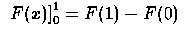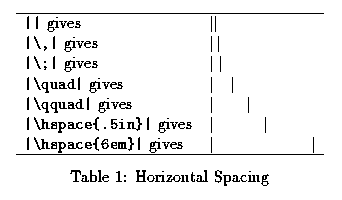# LaTeX Lesson 5 Typesetting Math in LaTeX

## Typing Mathematics

Let's take a look at how to handle some typical problems in using LaTeX to typeset mathematics. We have already looked at various math environments.

### Fractions

In order to get fractions in-line, you have a choice of using the solidus to get a fraction like 1/2, or of using the math mode command \frac{a}{b}, which will give a small fraction with numerator  a  and denominator  b  for in-line formulas. Using the "displayed fraction" command \dfrac{a}{b} gives an upright fraction, and makes the line a little larger vertically to provide sufficient vertical space for the displayed fraction. In a displaymath environment or  equation environment it is not necessary to use the \dfrac command; the command \frac{a}{b} will give an upright fraction, and the resulting line will also take extra vertical space.

Warning: note that the fraction command has two arguments, each enclosed in curly braces. Avoid non-LaTeX-style argument lists, like \frac{a,b}, an error that many beginners make when they first start typing LaTeX documents.

### Integrals

The integral sign is produced by the command \int, and one can attach the limits of integration by giving them as subscript and superscript to the command \int.

### Expanding Delimiters

LaTeX provides parenthesis-like symbols that will expand vertically so that they are tall enough to look well-matched to the height of the formula that they enclose. As an example, take the source code:
$\left| \frac{A+B}{3} \right|$

It produces a displayed version of the formula |(A+B)/3| in which the absolute value delimiters are expanded somewhat in length.

NOTE: The symbol | is located above the backslash on most keyboards. It works properly only in math environments. You can also use \vert to get the same result.

Now please look at the page of integral calculus provided by one of the two links below.

As an exercise, you are going to use LaTeX to typeset your own version of this page. [WORK IN PROGRESS]

In it we encounter the problem of using a single right delimiter, ], (with subscript and superscript attached) to indicate the difference of values of a function. LaTeX produces typeset copy in which the symbol ] is too small.

It is natural to think of using the idea introduced above for matching delimiters (such as []) to the text inside, and to try the code

$\int_0^1 \cdots dx = x \tan^{-1} x \right]$

but LaTeX complains if you try to use \right] without a corresponding \left] command earlier in the file. To get only a single right delimiter, say ], sized correctly you can use \big] or, if that is still too small, \bigg]; but you can also let LaTeX adjust the size of the delimiter by putting in a blank delimiter with the command \left., that is \left followed immediately by a period. For example,can be typeset by

\documentclass[12pt]{article}
\begin{document}
$\left. F(x) \right]_{0}^{1} = F(1) - F(0)$
\end{document}


### Spacing.

In math mode, the spaces in your source file are completely ignored by LaTeX with just two exceptions.
1. a space is one of the characters that indicates the end of a command.
2. you can insert a text box (see below) in which everything is in text mode.
For example,
$a b$
is typeset as ab.

Therefore, to achieve the appearance that you want, you may have to add or remove space. For example, in an integral, between the integrand and the differential it is usually better to add a "thinspace" \,.

Perhaps you will find this feature of LaTeX to be disagreeable, but eventually you should be able to appreciate the balance struck in LaTeX between convenience and the power to control the whitespace completely whenever it seems desirable. Here is a list of the most widely used commands for horizontal spacing.

• \,		(thinspace)
• \;		(thickspace)
• \quad   	(quadspace)
• \qquad  	(double quadspace)
• \!		(negative thinspace)
These commands work both in math environments and in text environments.

There are other ways of getting space. If you want 12 points of horizontal whitespace between two characters, you can get it by using the command \hspace{12pt} between them. The argument to the \hspace command can be specified in inches, for example, .5in if you want a half inch of horizontal whitespace. This command also works in both math and text environments. You may want to keep in mind that there are 72 points to the inch.

If you want whitespace at the beginning of a line, you need the variant form of the \hspace command, namely \hspace* because LaTeX generally swallows all space at the beginning of a line.

While we are on the subject of creating space, we should mention that there is a command \vspace which works very much like \hspace but creates vertical space in a document. This is needed, for instance, if you want to create vertical space for including a picture.

For simple vertical spacing one can use the predefined vertical spaces provided by the commands

• \smallskip
• \medskip
• \bigskip

It is sometimes convenient to use relative size units rather than absolute size units. You can refer to the relative size units in the current font by using the units em and ex. At one time, em and ex corresponded, respectively, to the widths of the letters M and X in whatever font was being used at that point. In LaTeX that is no longer true, but it is true that the size of these units will scale with the font, so that a change to a different font size will not disturb the proportions of the typeset material.

Here is some LaTeX code that will create a small table showing the effects of the various horizontal spacing commands.

\documentclass[12pt]{article}
\begin{document}
\begin{table}[ht]
\begin{center}
\begin{tabular}{ l l }
\hline
\verb=||= gives &  $||$ \\
\verb=|\,|= gives &  $|\,|$ \\
\verb=|\;|= gives &  $|\;|$ \\
\verb=|\quad|= gives &  $|\quad |$ \\
\verb=|\qquad|= gives & $|\qquad|$ \\
\verb=|\hspace{.5in}|= gives & $|\hspace{.5in}|$ \\
\verb=|\hspace{6em}|= gives & $|\hspace{6em}|$ \\
\hline
\end{tabular}
\caption{Horizontal Spacing}  %NOTE: this is within the center environment
\end{center}
\end{table}
\end{document}

It gives this table:You can also get horizontal space in a more flexible way by using LaTeX's text boxes.

### Boxes

You may already have wanted to write some text when you were in a math environment. There are several box constructions which do the job. Without using the amsmath package, one could use the command \makebox, which has a short form \mbox. These take
• an optional length argument, such as 3.5in (or cm or pt),
• an optional alignment argument
• l for flushed left (this is the default)
• r for flushed right,
• s for stretched,
• a text argument.
Thus, you could have a command
   \makebox[3in][r]{This is in a line box. }
This gives a 3 inch horizontal space in which the text "This is in a line box." is right justified. If you want to put a frame around your box, that is easy. Use
   \framebox[3in][r]{This is in a line box. }

### Exercise.

Your instructor has a handout with some material marked for you to typeset in LaTeX as an exercise, which you should do now. If your browser and platform are set up to use a PostScript viewer, like ghostview or ghostscript, then you can see the material by following the link: Material for Typesetting: PostScript version, or the link Material for Typesetting: Acrobat (PDF) version,.

### Tutorial at Cornell.

There is a nicely done tutorial at Cornell University. LaTeX tutorial at Cornell University

The part of the tutorial on errors in LaTeX is appropriate reading now since the most common error messages in LaTeX, besides misspelled commands, involve "overfull hbox" errors.

Go to their tutorial now.

### Matrices in LaTeX

Here is an example of some LaTeX code that will typeset a matrix.

\left[
\begin {array}{ccc}
9&13&17\\
\noalign{\medskip}
14&18&22
\end {array}
\right]


If you put this code inside a LaTeX displaymath environment, you will get the matrix typeset. (Since matrices are large, they are almost always set as displays.) Here are some points to observe about this code.

• The \left[ and \right] are delimiters of adjustable size that make the brackets around the matrix. If you want parentheses instead of square brackets, use \left( and \right). To make vertical bars for determinants, use \left\vert and \right\vert. You can also make curly braces via \left\{ and \right\}. (For curly braces, you need to put a backslash in front of the braces so that LaTeX realizes they are not LaTeX grouping symbols.)
• The LaTeX array environment has an argument, in this case ccc, that determines how the entries in each column are aligned. In this example, all entries are centered in their columns. If you change ccc to lrc, for example, then the entries in the first column will be left-aligned, the entries in the second column will be right-aligned, and the entries in the third column will be centered.
• The ampersand & character is used to separate entries in different columns.
• A double backslash \\ is used to terminate each row of the matrix except the last one.
• This example was produced by Maple. The source code \noalign{\medskip} in this example gives an extra space between the rows of the matrix. This extra space is not necessary and could be deleted.

(If you \usepackage{amsmath}, and you are willing to settle for centered entries in matrices, then you can replace the array environment with the matrix environment, which does better spacing. The amsmath package also has a pmatrix environment that has enclosing parentheses built in.)

Exercise: Typeset the following matrix.

      [ 47  41  31  33 ]
[                ]
[  1  12  27  12 ]
[                ]
[ 41   1  28  58 ]
[                ]
[ 35  24  23  34 ]


email: Martin Karel